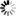Welcome to Central Library, SUST
Normal view

# Logical Foundations of Mathematics and Computational Complexity [electronic resource] : A Gentle Introduction / by Pavel Pudlák.

Material type:TextPublisher: Heidelberg : Springer International Publishing : Imprint: Springer, 2013Description: XIV, 695 p. 49 illus., 4 illus. in color. online resourceContent type:
• text
Media type:
• computer
Carrier type:
• online resource
ISBN:
• 9783319001197
Additional physical formats: Printed edition:: No titleDDC classification:
• 511.3 23
LOC classification:
• QA8.9-10.3
Online resources:
Contents:
Mathematician’s world -- Language, logic and computations -- Set theory -- Proofs of impossibility -- The complexity of computations -- Proof complexity -- Consistency, Truth and Existence -- References.
Summary: The two main themes of this book, logic and complexity, are both essential for understanding the main problems about the foundations of mathematics. Logical Foundations of Mathematics and Computational Complexity covers a broad spectrum of results in logic and set theory that are relevant to the foundations, as well as the results in computational complexity and the interdisciplinary area of proof complexity. The author presents his ideas on how these areas are connected, what are the most fundamental problems and how they should be approached. In particular, he argues that complexity is as important for foundations as are the more traditional concepts of computability and provability. Emphasis is on explaining the essence of concepts and the ideas of proofs, rather than presenting precise formal statements and full proofs. Each section starts with concepts and results easily explained, and gradually proceeds to more difficult ones. The notes after each section present some formal definitions, theorems and proofs. Logical Foundations of Mathematics and Computational Complexity is aimed at graduate students of all fields of mathematics who are interested in logic, complexity and foundations. It will also be of interest for both physicists and philosophers who are curious to learn the basics of logic and complexity theory.
Tags from this library: No tags from this library for this title.
Star ratingsAverage rating: 0.0 (0 votes)
No physical items for this record

Mathematician’s world -- Language, logic and computations -- Set theory -- Proofs of impossibility -- The complexity of computations -- Proof complexity -- Consistency, Truth and Existence -- References.

The two main themes of this book, logic and complexity, are both essential for understanding the main problems about the foundations of mathematics. Logical Foundations of Mathematics and Computational Complexity covers a broad spectrum of results in logic and set theory that are relevant to the foundations, as well as the results in computational complexity and the interdisciplinary area of proof complexity. The author presents his ideas on how these areas are connected, what are the most fundamental problems and how they should be approached. In particular, he argues that complexity is as important for foundations as are the more traditional concepts of computability and provability. Emphasis is on explaining the essence of concepts and the ideas of proofs, rather than presenting precise formal statements and full proofs. Each section starts with concepts and results easily explained, and gradually proceeds to more difficult ones. The notes after each section present some formal definitions, theorems and proofs. Logical Foundations of Mathematics and Computational Complexity is aimed at graduate students of all fields of mathematics who are interested in logic, complexity and foundations. It will also be of interest for both physicists and philosophers who are curious to learn the basics of logic and complexity theory.

There are no comments on this title.